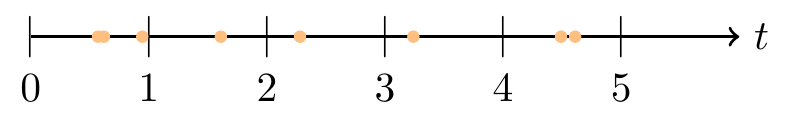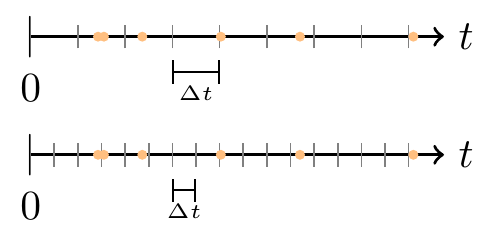# Lesson 17 Poisson Process

## Motivating Example

A Geiger counter is a device used for measuring radiation in the atmosphere. Each time it detects a radioactive particle, it makes a clicking sound. In the figure below, the orange points below indicate the times at which the Geiger counter detected a radioactive particle.Figure 17.1: Radioactive Particles Reaching a Geiger Counter

The radioactive particles hit the Geiger Counter at seemingly random and unpredictable times. In this lesson, we learn to make sense of the randomness.

## Theory

Radioactive particles are a classic example of a type of random process called the Poisson process. The Poisson process is used to model random events, called “arrivals”, over time.

Definition 17.1 (Poisson Process) A Poisson process of rate $$\lambda$$ is characterized by the following properties:

1. The number of arrivals in any interval $$(t_0, t_1)$$ follows a Poisson distribution with $$\mu = \lambda (t_1 - t_0)$$. That is, the parameter $$\mu$$ increases proportionally to the length of the interval.
2. The numbers of arrivals in non-overlapping intervals are independent.

These two properties allow us to calculate any probability of interest.

Example 17.1 (Geiger Counter) In San Luis Obispo, radioactive particles reach a Geiger counter according to a Poisson process at a rate of $$\lambda = 0.8$$ particles per second.

1. What is the probability that the Geiger counter detects 3 or more particles in the next 4 seconds?
2. What is the probability that the Geiger counter detects (exactly) 1 particle in the next second and 3 or more in the next 4 seconds?

Solution. .

1. The number of particles arriving at the detector in the next 4 seconds, $$X$$, follows a $$\text{Poisson}(\mu = 0.8 \cdot (4 - 0) = 3.2)$$ distribution by Property 1 (17.1). Therefore, the probability of detecting 3 or more particles is: \begin{align*} P(X \geq 3) &= 1 - P(X < 3) \\ &= 1 - f(0) - f(1) - f(2) \\ &= 1 - e^{-3.2} \frac{3.2^0}{0!} - e^{-3.2} \frac{3.2^1}{1!} - e^{-3.2} \frac{3.2^2}{2!} \\ &\approx .6201 \end{align*}
2. The joint probability $P(\text{1 particle in (0, 1)} \text{ AND } \text{3 or more particles in (0, 4)})$ involves two events that are not independent. However, we can rewrite the joint probability as: $P(\text{1 particle in (0, 1)} \text{ AND } \text{2 or more particles in (1, 4)}).$ Now, $$(0, 1)$$ and $$(1, 4)$$ are non-overlapping intervals, so the number of particles arriving in $$(0, 1)$$ is independent of the number of particles arriving in $$(1, 4)$$ by Property 2 (17.1). Therefore, by Theorem 7.1, we obtain their joint probability by multiplying their individual probabilities: $P(\text{1 particle in (0, 1)}) \cdot P(\text{2 or more particles in (1, 4)}).$

Now, we know from Property 1 (17.1) that

• the number of particles in $$(0, 1)$$ follows a $$\text{Poisson}(\mu = 0.8)$$ distribution, and
• the number of particles in $$(1, 4)$$ follows a $$\text{Poisson}(\mu = 2.4)$$ distribution.
If we represent the p.m.f.s of these two random variables by $$f_1$$ and $$f_2$$, respectively, then the probability is $f_1(1) \cdot \left(1 - f_2(0) - f_2(1) \right) = e^{-0.8} \frac{0.8^1}{1!} \cdot \left( 1 - e^{-2.4} \frac{2.4^0}{0!} - e^{-2.4} \frac{2.4^1}{1!} \right) \approx .2486.$

We can also calculate these probabilities with the aid of software:

from symbulate import *
Poisson(0.8 * (1 - 0)).pmf(1) * (1 - Poisson(0.8 * (4 - 1)).cdf(1))
## 0.24858997647262118

### Why Poisson?

Why does it make sense to assume that the number of arrivals on any interval follows a Poisson distribution? If we chop time up into tiny segments of length $$\Delta t$$, then the probability of an arrival on any one of these segments will be small—but there will be many such intervals.Figure 17.2: Chopping Up Time in a Poisson Process

If we further assume that arrivals are independent across these tiny segments, then the number of arrivals follows a binomial distribution, with large $$n$$ (because there are many segments) and small $$p$$ (because an arrival is unlikely to fall exactly in any given segment). We saw in Theorem 16.1 that the Poisson distribution is a good approximation to the binomial when $$n$$ is large and $$p$$ is small.

Technical Detail: In order for the number of arrivals to be binomial, each tiny segment must contain either 1 arrival or none; there cannot be 2 or more arrivals in any segment. Usually, if $$\Delta t$$ is so small that it is rare to get even 1 arrival in a segment, then it is essentially impossible to get 2 arrivals.

## Essential Practice

1. Packets arrive at a certain node on the university’s intranet at 10 packets per minute, on average. Assume packet arrivals meet the assumptions of a Poisson process.

1. Calculate the probability that exactly 15 packets arrive in the next 2 minutes.
2. Calculate the probability that more than 60 packets arrive in the next 5 minutes.
3. Calculate the probability that the next packet will arrive in within 15 seconds.
2. Small aircraft arrive at San Luis Obispo airport according to a Poisson process at a rate of 6 per hour.

1. What is the probability that exactly 5 small aircraft arrive in the first hour (after opening) and exactly 7 arrive in the hour after that?
2. What is the probability that fewer than 5 small aircraft arrive in the first hour and at least 10 arrive in the hour after that?
3. What is the probability that exactly 5 small aircraft arrive in the first hour and exactly 12 aircraft arrive in the first two hours?